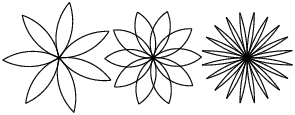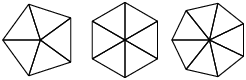Skip to main content

# 17.12: Exercises

Exercise $$\PageIndex{1}$$

Download the code in this chapter from http://thinkpython.com/code/polygon.py.

1. Write appropriate docstrings for polygon, arc and circle.
2. Draw a stack diagram that shows the state of the program while executing circle(bob, radius). You can do the arithmetic by hand or add print statements to the code.
3. The version of arc in Section 4.7 is not very accurate because the linear approximation of the circle is always outside the true circle. As a result, the turtle ends up a few units away from the correct destination. My solution shows a way to reduce the effect of this error. Read the code and see if it makes sense to you. If you draw a diagram, you might see how it works.Figure $$\PageIndex{1}$$: Turtle flowers.

Exercise $$\PageIndex{2}$$

Write an appropriately general set of functions that can draw flowers as in Figure 4.12.1.

Solution

http://thinkpython.com/code/flower.py, also requires http://thinkpython.com/code/polygon.py.Figure $$\PageIndex{2}$$: Turtle pies.

Exercise $$\PageIndex{3}$$

Write an appropriately general set of functions that can draw shapes as in Figure 4.12.2.

Solution

http://thinkpython.com/code/pie.py.

Exercise $$\PageIndex{4}$$

The letters of the alphabet can be constructed from a moderate number of basic elements, like vertical and horizontal lines and a few curves. Design a font that can be drawn with a minimal number of basic elements and then write functions that draw letters of the alphabet.

You should write one function for each letter, with names draw_a, draw_b, etc., and put your functions in a file named letters.py. You can download a “turtle typewriter” from http://thinkpython.com/code/typewriter.py to help you test your code.

Solution

http://thinkpython.com/code/letters.py, also requires http://thinkpython.com/code/polygon.py.

Exercise $$\PageIndex{5}$$

Read about spirals at http://en.Wikipedia.org/wiki/Spiral; then write a program that draws an Archimedian spiral (or one of the other kinds).

Solution

http://thinkpython.com/code/spiral.py.